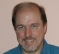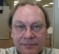## PLL in presence of noiseStarted by 7 years ago8 replieslatest reply 7 years ago202 views

Hi

Assume I have a vector of phases and I take a derivative from them and I obtained the frequencies however I am looking for to estimate the frequency of signal and by taking derivative I am obtaining a noisy vector of data, how would I extract the frequency of signal from my vector. I was thinking of using the mean command and this works very well when the data is not noisy however this method is not really robust to noise. Can anyone suggest me another technnique to extract the needed information from noisy data ?

[ - ]Have you done a web search on "frequency estimation"?   There are a number of techniques depending on what your unique constraints might be, but there's a lot of information already out there.

[ - ]There are many technique for frequency estimation but I am looking for to estimate it using PLL and I have a estimate of phase and i have a vector of derivative of phase which gives me frequency all I want is technique to give me an estimate of frequency in the noisy vector I get after differentiation.

[ - ]In another thread you mentioned obtaining frequency from a locked digital PLL, and I explained how to do that very accurately using the Phase Increment Register in the NCO and the integrator in the loop.

If the PLL is locked, the phase detector output and loop output will be only error quantities and will not have much to do with the signal or reference frequency unless the loop is poorly designed.

[ - ]Chess

I am not sure we understand the problem.

Differentiating always increases noise because it is a highpass filter. By taking the mean I assume that you mean a simple averaging filter, which is a primitive low-pass filter. You can improve this by using a properly designed low-pass filter.

However, you mention "PLL" in the title of you question. A PLL enables measuring the frequency in a different way from simple low-pass filter. A low pass LTI filter needs to be open to some constant bandwidth, on the other hand a PLL has some bandwidth, but it locks onto the signal (assuming the signal is within its capture range) and tracks it with this relatively small bandwidth. So, the way to recover frequency with a PLL is to observe the frequency of the VCO or NCO in the PLL.

Y(J)S

[ - ]Chess, Y(j)S makes a good point. As it turns out, there are digital differentiators that don't generate too much high-freq noise and their performance is pretty good so long as the input signal's predominate spectral energy is below Fs/4 Hz.

Having said that, you seem to have some real-valued sequence that represents the instantaneous phase of a complex-valued time sequence. If you differentiate that phase sequence you'll produce a real-valued sequence that's proportional to the frequency of your original complex-valued sequence. Is that what you really want to do? Are you, by any chance, trying to perform FM demodulation?

[ - ]Thanks Y(J)S, Rick and Slartibartfas. I can estimate the phase of reference signal accurately and now I would like to use my PLL to track the frequency (estimate the frequency) . I have a vector of phases and I take a derivative respect to them to obtain frequencies (As Rick you mentioned, that's exactly I am doing) and I realized simple operation like mean(signal) does not yield into good estimate. Y(J)S as you mentioned that is what I am doing with my PLL, I have a estimate of phase (output of VCO) then I am differentiating respect to time to get frequency . I am only taking mean because when I have vector of data (noisy signal) and I need to extract frequency (I am looking for estimate of frequency) average might seem to be good option. Assume I designed a better low pass filter then what I should with it ? I can smooth the signal with different low pass filter with different window size but after all I am looking for one number only.

[ - ]